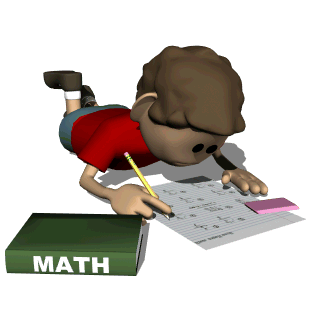• Math in FocusIn Grade 1, students will focus on four critical areas:

1. D
eveloping understanding of addition, subtraction, and strategies for addition and subtraction within 20

Students develop strategies for adding and subtracting whole numbers based on their prior work with small numbers. They use a variety of models, including discrete objects and length-based models (e.g., cubes connected to form lengths), to model add-to, take-from, put-together, take-apart, and compare situations to develop meaning for the operations of addition and subtraction, and to develop strategies to solve arithmetic problems with these operations. Students understand connections between counting and addition and subtraction (e.g., adding two is the same as counting on two). They use properties of addition to add whole numbers and to create and use increasingly sophisticated strategies based on these properties (e.g., “making tens”) to solve addition and subtraction problems within 20. By comparing a variety of solution strategies, children build their understanding of the relationship between addition and subtraction

2. Developing understanding of whole number
relationships and place value, including grouping in tens and ones

Students develop, discuss, and use efficient, accurate, and generalizable methods to add within 100 and subtract multiples of 10. They compare whole numbers (at least to 100) to develop understanding of and solve problems involving their relative sizes. They think of whole numbers between 10 and 100 in terms of tens and ones (especially recognizing the numbers 11 to 19 as composed of a ten and some ones). Through activities that build number sense, they understand the order of the counting numbers and their relative magnitudes.

3. D
eveloping understanding of linear measurement and measuring lengths as iterating length units

Students develop an understanding of the meaning and processes of measurement, including underlying concepts such as iterating (the mental activity of building up the length of an object with equal-sized units) and the transitivity principle for indirect measurement.

4. Reasoning about attributes of, and composing
and decomposing geometric shapes.

Students compose and decompose plane or solid figures (e.g., put two triangles together to make a quadrilateral) and build understanding of part-whole relationships as well as the properties of the original and composite shapes. As they combine shapes, they recognize them from different perspectives and orientations, describe their geometric attributes, and determine how they are alike and different, to develop the background for measurement and for initial understandings of properties such as congruence and symmetry.

Math in Focus:
• helps students build solid conceptual understanding through a focus on problem solving. Students will learn the "why" and the "how" through instruction, hands-on activities, and problem solving.
• is organized to teach fewer concepts at each level, but to teach them thoroughly. When a concept appears at a subsequent grade level, it is always at a higher or deeper level.
Concrete - Pictorial - Abstract

Our way of helping all students gain a strong conceptual understanding!